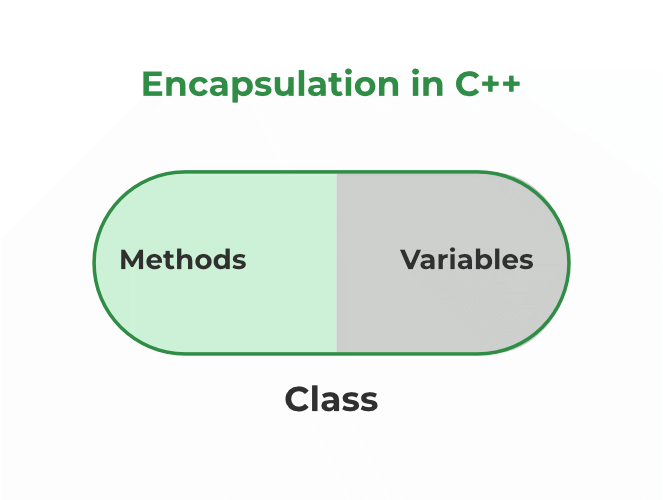GeeksforGeeks App
Open AppBrowser
Continue

# Encapsulation in C++

Encapsulation in C++ is defined as the wrapping up of data and information in a single unit. In Object Oriented Programming, Encapsulation is defined as binding together the data and the functions that manipulate them.

Consider a real-life example of encapsulation, in a company, there are different sections like the accounts section, finance section, sales section, etc. Now,

• The finance section handles all the financial transactions and keeps records of all the data related to finance.
• Similarly, the sales section handles all the sales-related activities and keeps records of all the sales.

Now there may arise a situation when for some reason an official from the finance section needs all the data about sales in a particular month.

In this case, he is not allowed to directly access the data of the sales section. He will first have to contact some other officer in the sales section and then request him to give the particular data.

This is what Encapsulation is. Here the data of the sales section and the employees that can manipulate them are wrapped under a single name “sales section”.

Two Important  property of Encapsulation

1. Data member and variables are declared private so to provide security.

2. Member function should be declared public so that anyone can’t change and work according to that function

For example if we give input , and output should be half of input

## C++

 `#include ``using` `namespace` `std;``class` `temp{``     ``int` `a;``  ``int` `b;``  ``public``:``  ``int` `solve(``int` `input){``    ``a=input;``    ``b=a/2;``    ``return` `b;``  ``}``};`` ` `int` `main() {``  ``int` `n;``  ``cin>>n;``  ``temp half;``  ``int` `ans=half.solve(n);``  ``cout<

## Features of Encapsulation

Below are the features of encapsulation:

1. We can not access any function from the class directly. We need an object to access that function that is using the member variables of that class.
2. The function which we are making inside the class must use only member variables, only then it is called encapsulation.
3. If we don’t make a function inside the class which is using the member variable of the class then we don’t call it encapsulation.
4. Increase in the security of data
5. It helps to control the modification of our data members.Encapsulation also leads to data abstraction. Using encapsulation also hides the data, as in the above example, the data of the sections like sales, finance, or accounts are hidden from any other section.

## C++

 `#include ``#include `` ` `using` `namespace` `std;`` ` `class` `Person {``  ``private``:``    ``string name;``    ``int` `age;``  ``public``:``    ``Person(string name, ``int` `age) {``      ``this``->name = name;``      ``this``->age = age;``    ``}``    ``void` `setName(string name) {``      ``this``->name = name;``    ``}``    ``string getName() {``      ``return` `name;``    ``}``    ``void` `setAge(``int` `age) {``      ``this``->age = age;``    ``}``    ``int` `getAge() {``      ``return` `age;``    ``}``};`` ` `int` `main() {``  ``Person person(``"John Doe"``, 30);`` ` `  ``cout << ``"Name: "` `<< person.getName() << endl;``  ``cout << ``"Age: "` `<< person.getAge() << endl;`` ` `  ``person.setName(``"Jane Doe"``);``  ``person.setAge(32);`` ` `  ``cout << ``"Name: "` `<< person.getName() << endl;``  ``cout << ``"Age: "` `<< person.getAge() << endl;`` ` `  ``return` `0;``}`

Output:

Name: John Doe
Age: 30
Name: Jane Doe
Age: 32

In C++, encapsulation can be implemented using classes and access modifiers.

Example:

## C++

 `// C++ program to demonstrate``// Encapsulation``#include ``using` `namespace` `std;`` ` `class` `Encapsulation {``private``:``    ``// Data hidden from outside world``    ``int` `x;`` ` `public``:``    ``// Function to set value of``    ``// variable x``    ``void` `set(``int` `a) { x = a; }`` ` `    ``// Function to return value of``    ``// variable x``    ``int` `get() { ``return` `x; }``};`` ` `// Driver code``int` `main()``{``    ``Encapsulation obj;``    ``obj.set(5);``    ``cout << obj.get();``    ``return` `0;``}`

Output

`5`

Explanation: In the above program, the variable x is made private. This variable can be accessed and manipulated only using the functions get() and set() which are present inside the class. Thus we can say that here, the variable x and the functions get() and set() are bound together which is nothing but encapsulation.

## C++

 `#include ``using` `namespace` `std;`` ` `// declaring class``class` `Circle {``    ``// access modifier``private``:``    ``// Data Member``    ``float` `area;``    ``float` `radius;`` ` `public``:``    ``void` `getRadius()``    ``{``        ``cout << ``"Enter radius\n"``;``        ``cin >> radius;``    ``}``    ``void` `findArea()``    ``{``        ``area = 3.14 * radius * radius;``        ``cout << ``"Area of circle="` `<< area;``    ``}``};``int` `main()``{``    ``// creating instance(object) of class``    ``Circle cir;``    ``cir.getRadius(); ``// calling function``    ``cir.findArea(); ``// calling function``}`

Output

```Enter radius
Area of circle=0```

## Role of Access Specifiers in Encapsulation

Access specifiers facilitate Data Hiding in C++ programs by restricting access to the class member functions and data members. There are three types of access specifiers in C++:

• Private
• Protected
• Public

By default, all data members and member functions of a class are made private by the compiler.

### Points to Consider

As we have seen in the above example, access specifiers play an important role in implementing encapsulation in C++. The process of implementing encapsulation can be sub-divided into two steps:

1. Creating a class to encapsulate all the data and methods into a single unit.
2. Hiding relevant data using access specifiers.